Processing ......FreeComputerBooks.com Links to Free Computer, Mathematics, Technical Books all over the World

A Friendly Introduction to Number Theory
Top Free Algorithms Books 🌠 - 100% Free or Open Source!
• Title A Friendly Introduction to Number Theory
• Author(s) Joseph H. Silverman
• Publisher: Pearson; 4 edition (January 28, 2012)
• Hardcover 432
• eBook PDF
• Language: English
• ISBN-10/ASIN: 0321816196
• ISBN-13: 978-0321816191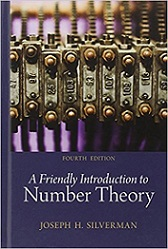Book Description

This book is an introductory text designed to entice non-math majors into learning some mathematics, while at the same time teaching them how to think mathematically. The exposition is informal, with a wealth of numerical examples that are analyzed for patterns and used to make conjectures. Only then are theorems proved, with the emphasis on methods of proof rather than on specific results.

It is designed to introduce readers to the overall themes and methodology of mathematics through the detailed study of one particular facet—number theory. Starting with nothing more than basic high school algebra, readers are gradually led to the point of actively performing mathematical research while getting a glimpse of current mathematical frontiers.

The writing is appropriate for the undergraduate audience and includes many numerical examples, which are analyzed for patterns and used to make conjectures. Emphasis is on the methods used for proving theorems rather than on specific results.

• Joseph H. Silverman is a Professor of Mathematics at Brown University. He received his Sc.B. at Brown and his Ph.D. at Harvard, after which he held positions at MIT and Boston University before joining the Brown faculty in 1988.
Reviews, Ratings, and Recommdations: Related Book Categories: Read and Download Links:Similar Books:
•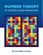Number Theory: In Context and Interactive (Karl-Dieter Crisman)

This is a textbook for an upper-level number theory course, with a clear vision to expose students to the connections to all areas of mathematics, and nearly every concept can be visualized or experimented with using the mathematics software SageMath.

•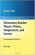Elementary Number Theory: Primes, Congruences, and Secrets

This is a book about prime numbers, congruences, secret messages, and elliptic curves that you can read cover to cover. Many numerical examples are given throughout the book using the SageMath mathematical software.

•An Introduction to the Theory of Numbers (Leo Moser)

This book, which presupposes familiarity only with the most elementary concepts of arithmetic (divisibility properties, greatest common divisor, etc.), is an expanded version of a series of lectures for graduate students on elementary number theory.

•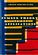Number Theory and Its Applications (Cheon Seoung Ryoo)

Number theory plays a fundamental and important role in mathematics and applied mathematics. This book is based on recent results in all areas related to number theory and its applications.

•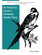An Introductory Course in Elementary Number Theory (Wissam Raji)

This book contains a useful introduction to important topics that need to be addressed in a course in number theory. Proofs of basic theorems are presented in an interesting and comprehensive way that can be read and understood even by non-majors.

•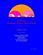Yet Another Introductory Number Theory Textbook (J. A. Poritz)

This introductory number theory textbook has a particular emphasis on connections to cryptology. It broaches the subject of these cryptologic algorithms to develop connections to the topic of number theory.

•Topology of Numbers (Allen Hatcher)

A textbook on elementary number theory from a geometric point of view, as opposed to the usual strictly algebraic approach. A fair amount of the book is devoted to studying Conway's topographs associated to quadratic forms in two variables.

•Magic Squares and Cubes (William Symes Andrews)

This book cover topics such as magic squares, magic cubes, the Franklin squares, magics and Pythagorean numbers, the theory of reversions, magic circles, spheres, and stars, and magic octahedroids, among other things.

•A Computational Introduction to Number Theory and Algebra

This introductory book emphasises algorithms and applications, such as cryptography and error correcting codes, and is accessible to a broad audience. The coverage includes the basics of number theory, abstract algebra and discrete probability theory.

•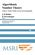Algorithmic Number Theory: Lattices, Curves, and Cryptography

This text provides a comprehensive introduction to algorithmic number theory for beginning graduate students, written by the leading experts in the field. It includes several articles that cover the essential topics in this area.

Book Categories
 :All CategoriesRecent BooksMiscellaneous BooksComputer LanguagesComputer ScienceData Science/DatabasesElectrical EngineeringJava and Java EE (J2EE)Linux and UnixMathematicsMicrosoft and .NETMobile ComputingNetworking and CommunicationsSoftware EngineeringSpecial TopicsWeb Programming
Other Categories